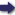(London :  Kegan Paul, Trench, Trübner & Co.,  1910.)

 Tools

## Search this bookPrev Page 51 Next``` CHAPTER LIII. 51 79,398,000 {rectd 79,497,000). Adding thereto 64,106 (i^ead 69,601), we get the sum 79,462,104 (orad 79,566,601). By dividing it by 210,902, we get the quotient T/^G (rectd 307), i.e. unardtra days, and a re¬ mainder of-J-f-ff-g-f (rectd -aW/oV)' *-^- 't^® avctmas. We subtract the iXnardtra days from the lunar days, written in the second place, and the remainder is the civil ctha,rganct, i.e. the sum of the civil days, viz. 23,684 (read 23,713). The method of the Po,ncct-Sid,dhdntikd of Varahami- Method^of hira is the following : " Take the years of the Sakakdlcc, siddhdntikd. subtract therefrom 427. Change the remainder into months by multiplying it by 12. Write down that number in two different places. Multiply the one number by 7 and divide the product by 228. The quotient is the number of ctdhimdsa months. Add them to the number written down in the second place, multiply the sum by 30, and add to the product the days which have elapsed of the current month. Write down the sum in two different places. Multiply the lower number by ii, add to the product 514, and divide page 228. the sum by 703. Subtract the quotient from the num¬ ber written in the upper place. The remainder you get is the number of the civil days." This, Varahamihira says, is the method of the Sid- dhanta of the Greeks. We exemplify this method in one of our gauge-years. Application From the years of the Sakakala we subtract 427. The me*thodto remainder, i.e. 526 years, is equal to 6312 months, yeir"^^' The corresponding number of adhimdsct months is 193 and a remainder of ^f. The sum of these months together with the other months is 6505, which are equal to 195,150 lunar days. The additions which occur in this method are required on account of the fractions of time which adhere to the epoch of the era in question. The multiplication by 7 is for the purpose of reducing the number to seventh parts. ```Prev Page 51 Next